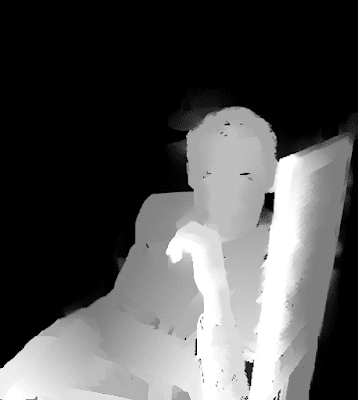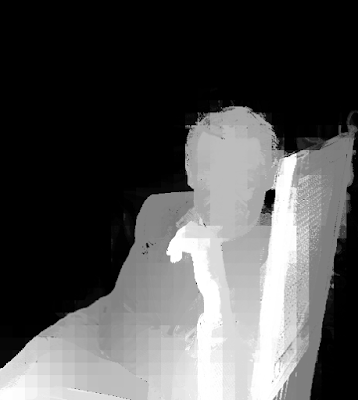## Tuesday, December 15, 2015

### 2D to 3D Conversion - Cary Grant

This is to compare Depth Map Automatic Generator 4 (DMAG4) which is based upon "Image segmentation using Scale-Space Random Walks" by R. Rzeszutek et al. and Depth Map Automatic Generator 9 (DMAG9) which is based upon "The Fast Bilateral Solver" by J. Barron et al. It's in the context of 2d to 3d image conversion, in other words, edge-aware densification (propagation) of a sparse depth map.Dense depth map obtained by DMAG4 using max number of iterations = 1000, number of scales = 1, level of graph connection within a scale = 2, and level of graph connection across scales= 1.

Because there are some areas that are totally black when they should not be, it would be a good idea to crank up the number of iterations.Dense depth map obtained by DMAG4 using max number of iterations = 2000, number of scales = 1, level of graph connection within a scale = 2, and level of graph connection across scales= 1.Dense depth map obtained by DMAG4 using max number of iterations = 3000, number of scales = 1, level of graph connection within a scale = 2, and level of graph connection across scales= 1.Dense depth map obtained by DMAG4 using max number of iterations = 4000, number of scales = 1, level of graph connection within a scale = 2, and level of graph connection across scales= 1.Dense depth map obtained by DMAG4 using max number of iterations = 5000, number of scales = 1, level of graph connection within a scale = 2, and level of graph connection across scales= 1.

Clearly, things get better as the number of iterations increases. Now, let's bring the number of iterations back down and increase the number of scales.Dense depth map obtained by DMAG4 using max number of iterations = 1000, number of scales = 2, level of graph connection within a scale = 2, and level of graph connection across scales= 1.Dense depth map obtained by DMAG4 using max number of iterations = 1000, number of scales = 3, level of graph connection within a scale = 2, and level of graph connection across scales= 1.

Clearly, as the number of scales increases, DMAG4 is less susceptible to noise and creates smoother gradations in the presence of noise/texture (back of the chair). Unfortunately, some object boundaries become blurrier (Cary's hair bleeds into the background).

As a finale, let's use a high number of iterations and high number of scales.Dense depth map obtained by DMAG4 using max number of iterations = 5000, number of scales = 3, level of graph connection within a scale = 2, and level of graph connection across scales= 1.

Not much a difference with the previous depth map, which means it's probably quite safe to reduce the number of iterations when increasing the number of scales.

Now, let's see what the challenger, DMAG9, can do.Dense depth map obtained by DMAG9 using Spatial sample rate = 4, Range (color) sample rate = 16, Lambda = 0.5, Hash table size = 10000, Number of PCG (Preconditioned Conjugate Gradient) iterations = 1000, Scale parameter of Geman-McClure function = 1.41, and Number of IRLS (Iteratively Reweighted Least Squares) iterations = 1.

Let's increase the range (color) sample rate to 32 in order to reduce the number of colors to (256/32)^3 = 512. This should reduce the number of un-propagated black pixels.Dense depth map obtained by DMAG9 using Spatial sample rate = 4, Range (color) sample rate = 32, Lambda = 0.5, Hash table size = 10000, Number of PCG (Preconditioned Conjugate Gradient) iterations = 1000, Scale parameter of Geman-McClure function = 1.41, and Number of IRLS (Iteratively Reweighted Least Squares) iterations = 1.

Some smoothness can be gained by increasing lambda.Dense depth map obtained by DMAG9 using Spatial sample rate = 4, Range (color) sample rate = 32, Lambda = 100, Hash table size = 10000, Number of PCG (Preconditioned Conjugate Gradient) iterations = 1000, Scale parameter of Geman-McClure function = 1.41, and Number of IRLS (Iteratively Reweighted Least Squares) iterations = 1.

To improve the behavior of DMAG9, it's usually a good idea to blur the reference image.Blurred version of the reference image. I used Gimp's Gaussian Blur (bandwidth = 5 pixels in both directions).

Alright, let's do another round of DMAG9 passes on this blurred reference image.Dense depth map obtained by DMAG9 using Spatial sample rate = 4, Range (color) sample rate = 16, Lambda = 0.5, Hash table size = 10000, Number of PCG (Preconditioned Conjugate Gradient) iterations = 1000, Scale parameter of Geman-McClure function = 1.41, and Number of IRLS (Iteratively Reweighted Least Squares) iterations = 1.Dense depth map obtained by DMAG9 using Spatial sample rate = 4, Range (color) sample rate = 32, Lambda = 0.5, Hash table size = 10000, Number of PCG (Preconditioned Conjugate Gradient) iterations = 1000, Scale parameter of Geman-McClure function = 1.41, and Number of IRLS (Iteratively Reweighted Least Squares) iterations = 1.Dense depth map obtained by DMAG9 using Spatial sample rate = 4, Range (color) sample rate = 32, Lambda = 100, Hash table size = 10000, Number of PCG (Preconditioned Conjugate Gradient) iterations = 1000, Scale parameter of Geman-McClure function = 1.41, and Number of IRLS (Iteratively Reweighted Least Squares) iterations = 1.

At this point in time, it looks like DMAG4 (Scale Space Random Walks) has the edge over DMAG9 (Fast Bilateral Solver) in the context of 2d to 3d image conversion. As far as DMAG4 is concerned, I am not at all convinced that Scale Space Random Walks (number of scales > 1) is that much better than plain Random Walks (number of scales = 1) but it's there as an option.

Update (12/16/2015):

After publishing this blog post, I realized that I had not played around with the spatial sample rate. So, let's go back to our "base" experiment before increasing the spatial sample rate in an effort to get rid of those "black" pixels. Note that it's a slightly different sparse depth map (modified around Cary's magnificent hair).Dense depth map obtained by DMAG9 using Spatial sample rate = 4, Range (color) sample rate = 16, Lambda = 0.5, Hash table size = 10000, Number of PCG (Preconditioned Conjugate Gradient) iterations = 1000, Scale parameter of Geman-McClure function = 1.41, and Number of IRLS (Iteratively Reweighted Least Squares) iterations = 1.Dense depth map obtained by DMAG9 using Spatial sample rate = 8, Range (color) sample rate = 16, Lambda = 0.5, Hash table size = 10000, Number of PCG (Preconditioned Conjugate Gradient) iterations = 1000, Scale parameter of Geman-McClure function = 1.41, and Number of IRLS (Iteratively Reweighted Least Squares) iterations = 1.Dense depth map obtained by DMAG9 using Spatial sample rate = 16, Range (color) sample rate = 16, Lambda = 0.5, Hash table size = 10000, Number of PCG (Preconditioned Conjugate Gradient) iterations = 1000, Scale parameter of Geman-McClure function = 1.41, and Number of IRLS (Iteratively Reweighted Least Squares) iterations = 1.

If I had the patience, I would then proceed to apply a bit of edge-aware smoothing, for instance, Edge Preserving Smoothing 7 (EPS7). In the end, DMAG9 appears to be doing as good a job as DMAG4 as long as you're using the right parameters.

#### 1 comment:

1.Nice!! but check out this 3D "photo" – rotate and zoom with mouse or touch

http://www.libak.dk/3Dscanning/examples/game1.html